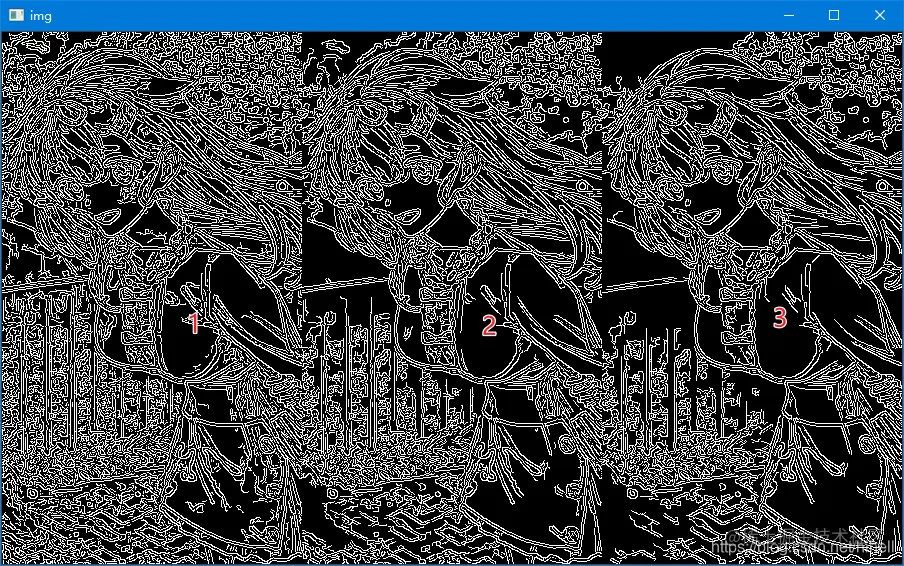# Python opencv Canny edge detection knowledge supplement

2022-01-30 07:22:49

Little knowledge , Great challenge ！ This article is participating in 「 A programmer must have a little knowledge 」 Creative activities

This article has participated in  「 Digging force Star Program 」 , Win a creative gift bag , Challenge creation incentive fund .

Python OpenCV 365 Day study plan , Go into the field of image with the eraser . This blog is the third in this series 47 piece .

stay Canny Edge extraction related knowledge learning , Image processing part 32 blog In this blog , We're right `Canny` Basic learning of edge detection , Today's article is mainly used to supplement it , Of course, knowledge is not difficult ,1 You can learn in an hour .

## Canny Edge detection process

Refer to the most published processes on the Internet

1. Gaussian blur denoising ;
2. Calculate the gradient amplitude and direction of the image , What is commonly used here is Sobel operator ;
3. Non maximum suppression , The simple understanding is “ Thin edge ”;
4. Double threshold detection , Also called lag threshold .

Follow the above steps to translate , You can write one Canny A simple case

``````import cv2 as cv
import numpy as np

src = cv.imread("./t7.jpg", 0)
#  Gaussian blur
gaussian = cv.GaussianBlur(src, (3, 3), 0)
ret, thresh = cv.threshold(gaussian, 0, 255, cv.THRESH_BINARY | cv.THRESH_OTSU)
#  Calculate the gradient amplitude and direction of the image , What is commonly used here is  Sobel  operator ;
sobel_x = cv.Sobel(thresh, cv.CV_16SC1, 1, 0)
sobel_y = cv.Sobel(thresh, cv.CV_16SC1, 0, 1)
sobel_x = cv.convertScaleAbs(sobel_x)
sobel_y = cv.convertScaleAbs(sobel_y)
sobel_xy = cv.addWeighted(sobel_x, 0.5, sobel_y, 0.5, 0)

canny1 = cv.Canny(sobel_xy, 50, 150)

image = np.hstack((thresh, canny1))
cv.imshow('img', image)
cv.waitKey(0)
cv.destroyAllWindows()
Copy code ``````There are some things to explain in the code , Double threshold detection adopts high and low threshold detection , High threshold usage `maxVal`、 Low threshold use `minVal`, There are the following conclusions .

1. Gradient value > maxVal , Boundary preservation ;
2. minVal < Gradient value < maxVal when , If the pixel gradient value is on the boundary , Retain , otherwise , Abandon ;
3. Gradient value < minVal, Abandon .

The vernacular of the above three points , Very easy to remember , If you want more boundaries , The small `minVal` value , Otherwise, turn it up .

In the actual operation, I also try to find a problem , If binarization is performed before edge extraction , The resulting image is less affected by the above parameters , For example, the following code , Double threshold setting different values , The results obtained are basically the same .

``````import cv2 as cv
import numpy as np

src = cv.imread("./t77.jpg", 0)
#  Gaussian blur
gaussian = cv.GaussianBlur(src, (3, 3), 0)
ret, thresh = cv.threshold(gaussian, 0, 255, cv.THRESH_BINARY | cv.THRESH_OTSU)
#  Calculate the gradient amplitude and direction of the image , What is commonly used here is  Sobel  operator ;
sobel_x = cv.Sobel(thresh, cv.CV_16SC1, 1, 0)
sobel_y = cv.Sobel(thresh, cv.CV_16SC1, 0, 1)
sobel_x = cv.convertScaleAbs(sobel_x)
sobel_y = cv.convertScaleAbs(sobel_y)
sobel_xy = cv.addWeighted(sobel_x, 0.5, sobel_y, 0.5, 0)

canny1 = cv.Canny(sobel_xy, 10, 30)
canny2 = cv.Canny(sobel_xy, 30, 90)
canny3 = cv.Canny(sobel_xy, 50, 150)
image = np.hstack((canny1, canny2,canny3))
cv.imshow('img', image)
cv.waitKey(0)
cv.destroyAllWindows()

Copy code ``````If you remove binarization , There is a clear difference in the edge of the image , Now I understand why I was just explaining Canny Edge extraction steps , There is no binarization step , It seems superfluous .

``````#  Gaussian blur
gaussian = cv.GaussianBlur(src, (3, 3), 0)
# ret, thresh = cv.threshold(gaussian, 0, 255, cv.THRESH_BINARY | cv.THRESH_OTSU)
#  Calculate the gradient amplitude and direction of the image , What is commonly used here is  Sobel  operator ;
Copy code ``````There's a little problem , It was found during the test , The edges obtained by using the following two pieces of code are inconsistent , As follows , You can test separately . The difference is in the way 1 adopt Sobel Operators calculate x Direction and y Directional gradient , After using the merged image Canny edge detection , The resulting edge is a double line , Method 2 Better effect, ideal .

``````#  Method 1
import cv2 as cv
import numpy as np

src = cv.imread("./t77.jpg", 0)
#  Gaussian blur
gaussian = cv.GaussianBlur(src, (3, 3), 0)

#  Calculate the gradient amplitude and direction of the image , What is commonly used here is  Sobel  operator ;
sobel_x = cv.Sobel(gaussian, cv.CV_16SC1, 1, 0)
sobel_y = cv.Sobel(gaussian, cv.CV_16SC1, 0, 1)
sobel_x = cv.convertScaleAbs(sobel_x)
sobel_y = cv.convertScaleAbs(sobel_y)
sobel_xy = cv.addWeighted(sobel_x, 0.5, sobel_y, 0.5, 0)

canny1 = cv.Canny(sobel_xy, 50, 150)
# canny2 = cv.Canny(sobel_x,sobel_y, 50, 150)
image = np.hstack((gaussian, canny1))
cv.imshow('img', image)
cv.waitKey(0)
cv.destroyAllWindows()

###  Method 2
import cv2 as cv
import numpy as np

src = cv.imread("./t77.jpg", 0)
#  Gaussian blur
gaussian = cv.GaussianBlur(src, (3, 3), 0)

#  Calculate the gradient amplitude and direction of the image , What is commonly used here is  Sobel  operator ;
sobel_x = cv.Sobel(gaussian, cv.CV_16SC1, 1, 0)
sobel_y = cv.Sobel(gaussian, cv.CV_16SC1, 0, 1)

canny2 = cv.Canny(sobel_x, sobel_y, 50, 150)
image = np.hstack((gaussian, canny2))
cv.imshow('img', image)
cv.waitKey(0)
cv.destroyAllWindows()
Copy code ``````Unfortunately Non maximum suppression The learning of relevant knowledge , It needs to be postponed , The current knowledge reserve is insufficient , It's hard to learn , We'll see you later .

## Eraser bars

I hope today's 1 You get something in an hour , I'll see you on our next blog ~